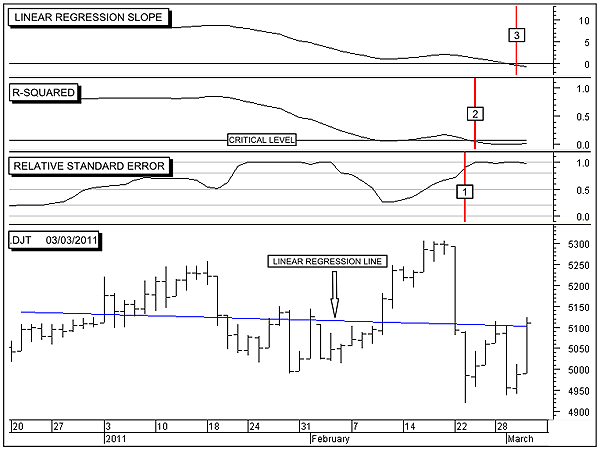HOT TOPICS LIST

INDICATORS LIST

LIST OF TOPICS

# Transportation Five-Step Reversal

03/04/11 09:23:17 AM
by Alan R. Northam

Within the study of statistical analysis, I have defined five steps to a reversal in trend. In this study I use the Dow Jones Transportation Average as the subject of analysis in identifying the steps that have been taken toward a trend reversal.

Security:   .DJT
Position:   N/A

 The Dow Jones Transportation Average (DJTA) remains in a long-term uptrend. In this study we will be looking at 50-day time frame to determine when the trend of the transportation average might be changing. In Figure 1, I show the daily bar chart for the .DJT in the bottom panel. However, in this study I want to concentrate on the three indicators in the panels above the daily bar chart. The first indicator I want to look at is the relative standard error index in the panel just above the daily price bar panel. The relative standard error index is a measure of volatility. The first step to a trend reversal normally occurs when volatility rises to a high level. Looking at the normal standard error indicator often found in charting programs, it is a difficult matter to make a decision as to what constitutes high volatility. As a result I have developed the relative standard error index shown. Volatility is considered to be above average when the relative standard error index (RSEI) moves above 0.5 and is considered to be high when the RSEI moves above 0.8. Extreme volatility is indicated when the RSEI reaches 1.0, the highest level attainable for the index. As can be seen in Figure 1, the .DJT moved above 0.8 on February 23, completing the first step to a reversal.FIGURE 1: .DJT, DAILY. The bottom panel shows the daily bar chart for the DJTA. The top window shows the linear regression slope indicator, the second lower window shows that R-squared indicator, and the third lower window the relative standard error index (RSEI). Graphic provided by: MetaStock. The next indicator I want to look at is the R-squared indicator. R-squared is a statistical mathematical calculation for the coefficient of determination. R-squared ranges from zero to 1, with zero meaning that the movement of stock prices are completely random and 1 meaning that all the movement of price is trending and there is no randomness involved. Thus, when price is completely trending without any randomness, the price movement is considered to be in a strong trend. The more randomness is involved, the weaker the trend is.There is a second part to the R-squared indicator, and that is the critical level. The critical level is a moving level, depending upon the time frame being measured. In statistics, the critical level has been determined to represent a line that determines when there is a 95% probability that a trend is strong enough to continue.As we can see in Figure 1, the .DJT has moved below this critical level and is extremely close to zero. This means that the price movement of the DJTA is completely random and without trend. Another way of saying this is that the trend is so weak, there is no trend. Note that the .DJT moved below its critical level on February 24, completing step 2 of the five steps to a reversal. The next indicator I want to look at is the linear regression slope indicator. This indicator is part of the equation that determines the linear regression line in statistics and is the part that determines the slope of the regression line. When this indicator is above its zero line, it indicates that the linear regression line is moving upward and is a measure of the direction of the trend, which is upward. When the indicator is below its zero line, it is indicating that the direction of the trend is downward. As can be seen in Figure 1, the linear regression slope indicator moved below its zero line on March 2, signaling a reversal in trend from up to down and completing step 3 of a five-step process to a reversal.As shown in Figure 1, steps 4 and 5 have not been completed. To complete the reversal from an uptrend to a downtrend, step 4 occurs when the R-squared indicator moves back above its critical level. This will indicate that there now exists a 95% probability that the new downtrend will continue. Until R-squared moves above its critical level, there is a high probability that the new downward trend will fail. Step 5 then occurs when the relative standard error index moves below 0.2. This will signal low volatility. Low volatility normally occurs once price starts to trend and the randomness of price (volatility) subsides. Low volatility is thus a confirmation that the new trend is now under way. In conclusion, the DJTA has now reversed its trend from up to down. However, at this point the new downtrend is extremely weak and prone to failure. For the new downtrend to succeed, we need to see the R-squared indicator move above its critical level, and we need to see the relative standard error index move below 0.2. All five steps are necessary for a successful trend reversal to have occurred.

Alan R. Northam

Alan Northam lives in the Dallas, Texas area and as an electronic engineer gave him an analytical mind from which he has developed a thorough knowledge of stock market technical analysis. His abilities to analyze the future direction of the stock market has allowed him to successfully trade of his own portfolio over the last 30 years. Mr. Northam is now retired and trading the stock market full time. You can reach him at inquiry@tradersclassroom.com or by visiting his website at http://www.tradersclassroom.com. You can also follow him on Twitter @TradersClassrm.

 Comments or Questions? Article Usefulness 5 (most useful) 4 3 2 1 (least useful)